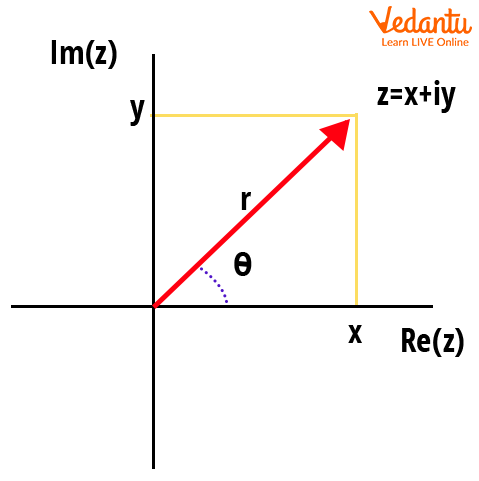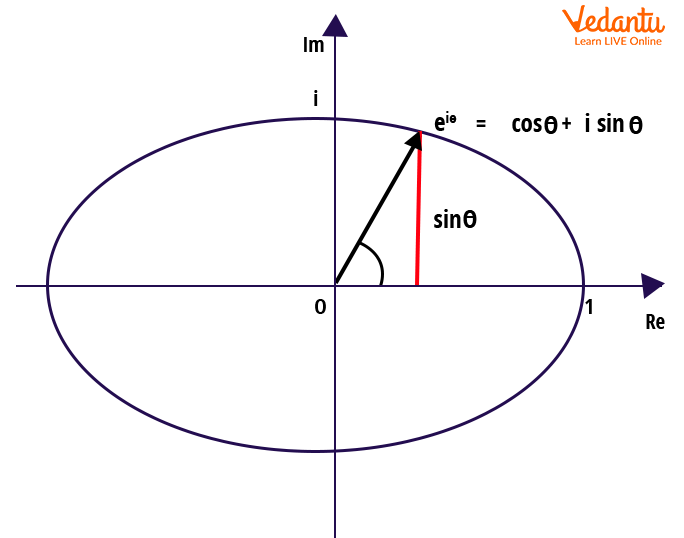Courses
Courses for Kids
Free study material
Free LIVE classes
More

# Polar and Euler Form of Complex Number for JEELIVE
Join Vedantu’s FREE Mastercalss

## The Polar and Euler Form of a Complex Number

A complex number can be represented as a vector in an argand plane or the Gaussian plane. A complex number can be represented in rectangular or Cartesian form, polar form and Euler Form. In the Cartesian or rectangular form, the complex number is denoted z=x+iy, where i is the imaginary part. In the polar form, the complex numbers are represented as a combination of modulus and argument.

The Modulus of a complex number is nothing but its distance from the origin in the argand plane. In other words, we can also define the modulus of a complex number as a point on the argand plane and its distance from the origin. The argument of a complex number on the other hand is defined as the angle between the positive real axis of the argand plane and the line representing the complex number. Talking about the Euler form of a complex number it is nothing but a relationship between exponential and trigonometric functions.

## Polar Form of Complex Number

As stated above a complex number can be represented in different forms. One of these is the polar form. In polar form, a complex number is represented as a combination of a modulus and an argument.## Polar Representation of Complex Numbers

Mathematically, the polar form of a complex number is represented as, z = r (cos(θ) + i sin (θ) )where r represents the modulus and is the argument. If a complex number is denoted as z= x+iy, then $r=\sqrt{x^{2}+y^{2}}$ and $\theta=\tan ^{-1}\left(\dfrac{y}{x}\right)$

The argument is calculated as follows :

• If z lies in the first or fourth quadrant, then $\theta=\tan ^{-1}\left(\dfrac{y}{x}\right)$

• If z lies in the second quadrant, then $\theta=\tan ^{-1}\left(\dfrac{y}{x}\right)+180$

• If z lies in the third quadrant, then $\theta=\tan ^{-1}\left(\dfrac{y}{x}\right)-180$

## Complex Number Euler Form

We know that a complex number can be represented in Cartesian or rectangular form as x+iy, where x and y are the real and imaginary parts of the complex number.  But we have also seen above that a complex number can also be represented in polar form as $r(\cos \theta+i \sin \theta)$, where r is the magnitude of the complex number. It can also be defined as the distance of a point of a complex number in the argand plane from the origin. $\theta$ as we know is the argument or the angle formed with the real axis.

There is another form in which a complex number can be represented which is the Euler form or Euler rule. The Euler form is also sometimes referred to as the complex exponential form and is expressed as:A Pictorial Representation of the Euler’s Form.

## Euler’s Formula Examples

1. Find the value of $e^{\dfrac{i \pi}{ 6}}$

Solution:

Given $e^{\dfrac{i \pi}{ 6}}$

Now using the Using Euler’s formula, we have

\begin{align} &e^{i x}=\cos x+i \sin x \\ &e^{\dfrac{i \pi}{ 6}}=\cos \dfrac{\pi}{6}+i \sin \dfrac{\pi}{6} \\ &e^{\dfrac{i \pi} {6}}=0.866+i \times 1 \\ &e^{\dfrac{i \pi } {6}}=i+0.866 \end{align}

2. Find the value of $e^{\dfrac{i \pi}{ 7}}$

Solution:

Given $e^{\dfrac{i \pi}{ 7}}$

Now using the Using Euler’s formula, we have

\begin{align} &e^{ix}=\cos x+i \sin x \\ &e^{\dfrac{i \pi}{ 7}}=\cos \dfrac{\pi}{7}+i \sin \dfrac{\pi}{7} \\ &e^{\dfrac{i \pi}{ 7}}=0.9+i \times 1 \\ &e^{\dfrac{i \pi} {7}}=i+0.9 \end{align}

## Euler’s Formula Derivation

In complex numbers, Euler’s formula bridges the gap between exponential and trigonometric functions. For example, if we have a complex number as z= a+ib, then according to Euler’s rule or formula we can say that = cos z + sin iz.

A simple and straightforward proof of Euler's formula is given by the power series. It entails expanding this power series in terms of exponents, sin and cosine functions to finally conclude Euler's identity.

We can expand cos z and sin z as below:

\begin{align} &\cos (z)=1-\dfrac{x^{2}}{2 !}+\dfrac{x^{4}}{4 !} \\ &\sin (z)=1-\dfrac{x^{3}}{3 !}+\dfrac{x^{5}}{5 !} \\ &\cos (z)+i \sin (z)=1+i z-\dfrac{x^{2}}{2 !}-\dfrac{x^{3}}{3 !}+\dfrac{x^{4}}{4 !} \end{align}

$e^{i z}$

### Convert into Polar FormPolar Form of A Complex Number

With complex numbers, we make an assumption that r which is the magnitude is always positive whereas the argument can be positive or negative.

Now coming to the derivation, to represent complex numbers in exponential form, we will need to use Euler's formula which is given by:

$r^{i \theta}=\cos (\theta)+i \sin (\theta)$------(1)

We represent the complex number z=a+bi in polar form as $z=r(\cos \theta+i \sin \theta)$-----(2)

Thus, by substituting (1) in (2) we get, $z=re^{i\theta}$, where $\theta$ is in radians.

Thus, we get the exponential form of a complex number.

## Conclusion

The article presents a thorough overview of the polar form and the Euler form of the complex numbers. It also gives a thorough review of Euler’s form derivation followed by some examples. The article also discusses the conversion of Euler’s form into polar form. Complex numbers are one of the important topics. It must be studied thoroughly as it simplifies mathematical calculations.

Last updated date: 22nd Sep 2023
Total views: 128.1k
Views today: 2.28k

## FAQs on Polar and Euler Form of Complex Number for JEE

1. How to write the Cauchy-Riemann CR Equation in polar form?

The partial derivatives of u and v are used in the Cauchy-Riemann equations to allow us to accomplish two things: first, check if f has a complex derivative, and second, compute that derivative. We begin by presenting the equations in the form of a theorem. The Cauchy-Riemann equations are nothing but partial differential equations that must be satisfied by the real and imaginary parts of a complex number or complex-valued function.

The Cauchy-Riemann equations in Polar form are denoted as:

$f\left(r e^{i \theta}\right)=u(r, \theta)+i v(r, \theta)$

2. How to add complex numbers in polar form?

Suppose we have two complex numbers one is in rectangular form and another one is in polar form. We need to first represent them both in polar form.

Let 4∠20° , 3 + 6i are the two complex numbers.

First, we will convert 4∠20°   into a rectangular form.

4∠20°

equals x + iy

Hence, x = 4 cos 20° = 3.75

y = 4 sin 20°  = 1.368

So, 7∠20°

equals 3.75+ i 1.368

Therefore, when we add any two given complex numbers, we get;

(3 + i5)+ (3.75 + i 1.368) = 7.5 + I 10.36

Now again we have to convert the resulting complex number into polar form. Thus we find the modulus and argument of the resulting number.

Therefore, the Modulus is equal to; $r=|z|=\sqrt{\left(x^{2}+y^{2}\right)}$ and we find the argument by

$\theta=\tan ^{-1}(\dfrac{y} {x})$

Finally, we get the required complex number in the form $r \sqrt{\theta}$